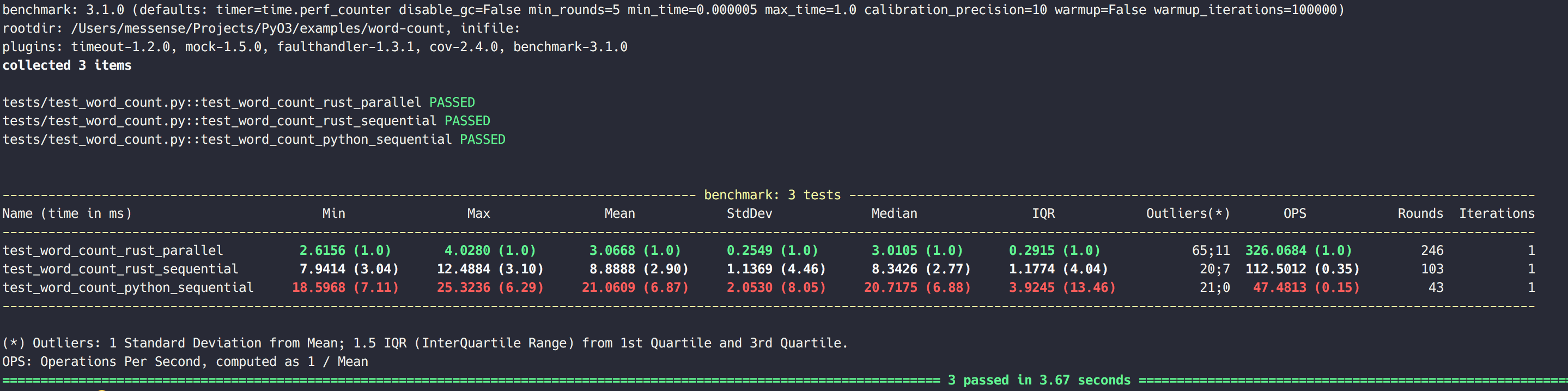# Parallelism

CPython has the infamous GIL (Global Interpreter Lock), which prevents developers from getting true parallelism when running pure Python code. With PyO3, you can release the GIL when executing Rust code to achieve true parallelism.

The `Python::allow_threads` method temporarily releases the GIL, thus allowing other Python threads to run.

``````impl Python {
pub fn allow_threads<T, F>(self, f: F) -> T where F: Send + FnOnce() -> T {}
}
``````

Let's take a look at our word-count example, where we have a `wc_parallel` function that utilizes the rayon crate to count words in parallel.

``````fn wc_parallel(lines: &str, search: &str) -> i32 {
lines.par_lines()
.map(|line| wc_line(line, search))
.sum()
}
``````

Then in the Python bridge, we have a function `search` exposed to the Python runtime which calls `wc_parallel` inside a closure passed to `Python::allow_threads` to enable true parallelism:

``````#[pymodule]
fn word_count(py: Python, m: &PyModule) -> PyResult<()> {

#[pyfn(m, "search")]
fn search(py: Python, path: String, search: String) -> PyResult<i32> {
let mut file = File::open(path)?;
let mut contents = String::new();

let count = py.allow_threads(move || wc_parallel(&contents, &search));
Ok(count)
}

Ok(())
}
``````

## Benchmark

Let's benchmark the `word-count` example to verify that we did unlock true parallelism with PyO3. We are using `pytest-benchmark` to benchmark three word count functions:

The benchmark script can be found here, then we can run `pytest tests` to benchmark them.

On MacBook Pro (Retina, 15-inch, Mid 2015) the benchmark gives: